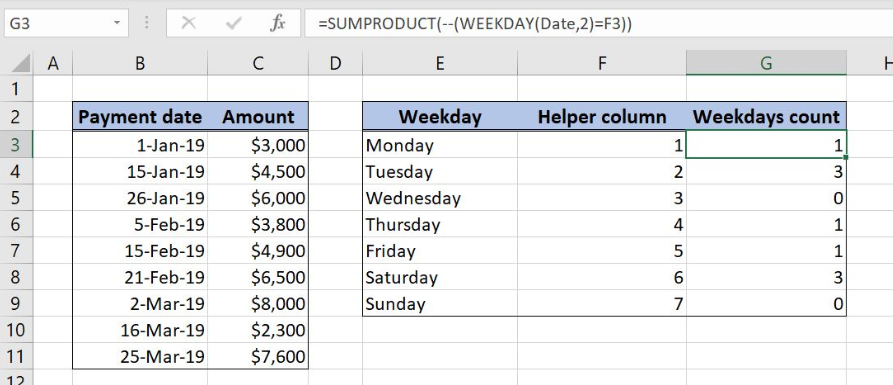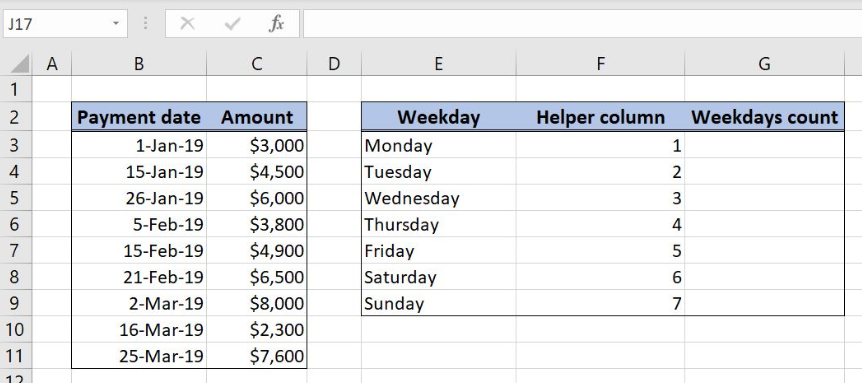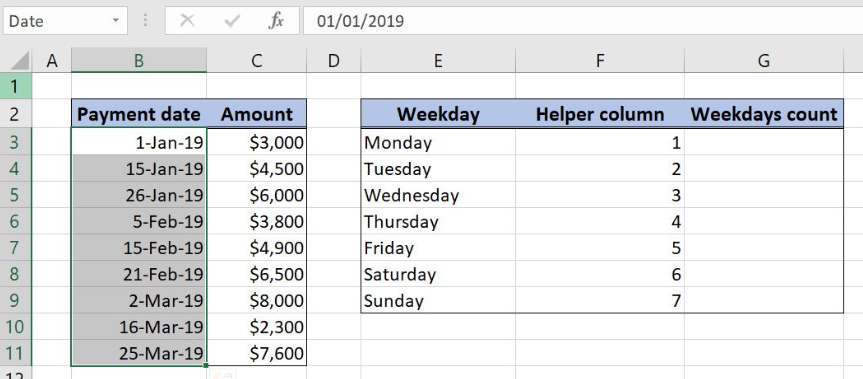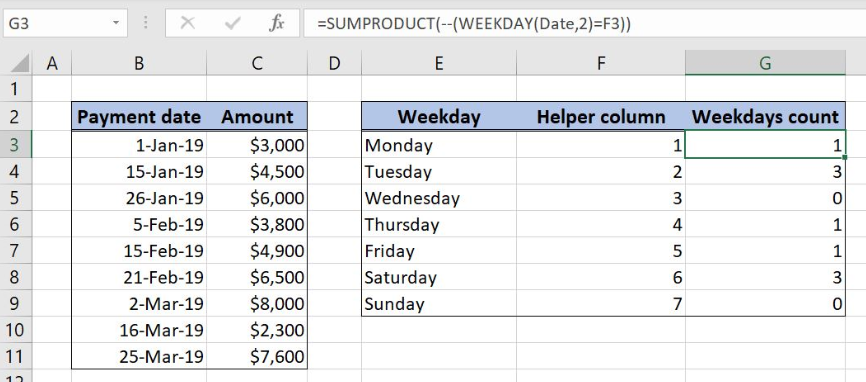Get instant live expert help with Excel or Google Sheets“My Excelchat expert helped me in less than 20 minutes, saving me what would have been 5 hours of work!”

#### Post your problem and you’ll get expert help in seconds.

Your message must be at least 40 characters
Our professional experts are available now. Your privacy is guaranteed.

# How to Count Dates by Day of the Week in Excel

With the combination of SUMPRODUCT and WEEKDAY function Excel allows us to count the number of weekdays within a date range. This step by step tutorial will assist all levels of Excel users in counting the dates by day of the week.Figure 1. Count weekdays in a Date range

## Syntax of the SUMPRODUCT Formula for Counting With Criteria

`=SUMPRODUCT(--(Cell_range=Criteria))`

The parameters of the SUMPRODUCT function for counting with criteria are:

• Cell_range – a cell range where we want to count the data
• Criteria – a criteria for counting

## Syntax of the WEEKDAY Formula

`=WEEKDAY(date,[return_type])`

The parameters of the WEEKDAY function are:

• date – date value from which we want to get the weekday
• return_type – indicates the start day of the week (1- week starts from Sunday to Saturday, 2-weeks starts from Monday to Sunday)

## Setting up Our Data for Counting the Dates by Weekday

Our first table consists of 2 columns: “Payment date” (column B) and “Amount” (column C). The second one has three columns: “Weekday” (Column E), “Helper column” (Column F) and “Weekdays count” (Column G). The idea is to count the number of the weekdays from “Payment date” column and to place the result in the column G.Figure 2. Table structure for counting weekdays

## SUMPRODUCT and WEEKDAY Function for Weekdays Counting

We want to get the number of weekdays from the column “Payment date”. In order to make the formula more clear, we will create a named range Date for cell range B3:B12.

To create a named range we should follow the steps:

• Select the cell range that should be named
• Click on the name box in Excel
• Write the name for the cell range and press enterFigure 3. Creating a named range Date for column “Payment date”

The formula for counting the weekdays within a Date range looks like:

`=SUMPRODUCT(--(WEEKDAY(Date,2)=F3))`

The cell_range in the SUMPRODUCT function is the formula part WEEKDAY(Date,2) while the criterion is the weekday number in the cell F3. In WEEKDAY function date is a named range Date, while the return_type is 2.

To apply the SUMPRODUCT function for counting the weekdays we need to follow these steps:

• Select cell G3 and click on it
• Insert the formula: `=SUMPRODUCT(--(WEEKDAY(Date,2)=F3))`
• Press enter
• Drag the formula down to the other cells in the column by clicking and dragging the little “+” icon at the bottom-right of the cell.Figure 4. Count Dates by Day of Week using SUMPRODUCT and WEEKDAY function

Formula WEEKDAY(Date,2 ) converts each date from the Date named range to the weekday number: `{2;2;6;2;5;4;6;6;1}`.

This array is then compared with the value 1 from the cell F3. Output array after comparison looks like: `{FALSE;FALSE;FALSE;FALSE;FALSE;FALSE;FALSE;FALSE;TRUE}`.

Double negative sign “–” in SUMPRODUCT function translates TRUE and FALSE values into numbers 1 and 0: `{0;0;0;0;0;0;0;0;1}`. The final result is the number 1, a sum of all final array numbers.

Most of the time, the problem you will need to solve will be more complex than a simple application of a formula or function. If you want to save hours of research and frustration, try our live Excelchat service! Our Excel Experts are available 24/7 to answer any Excel question you may have. We guarantee a connection within 30 seconds and a customized solution within 20 minutes.

### Did this post not answer your question? Get a solution from connecting with the expert.Another blog reader asked this question today on Excelchat:
Solution examplesUse the Vlookup Function to complete the "employee" column of table 2. Use "job Id" from table 2 as your lookup_value(s) and table 1 as your reference.
Solved by C. H. in 16 minsIf a cell in another sheet is populated I need a vlookup done. If the cell is not populated I need the cell to return blank.
Solved by T. D. in 60 minsI am trying to make a chart that turns a week range red if nothing is entered in the range. If something is entered then I would like it to turn green. Please Help
Solved by E. U. in 43 minsI need a check box to show/hide an answer of an if function
Solved by Z. U. in 23 minsI need a formula to compare the data in two columns and then export the mismatched data in the 3rd column
Solved by S. Q. in 20 mins## Subscribe to Excelchat.coAnother blog reader asked this question today on Excelchat: